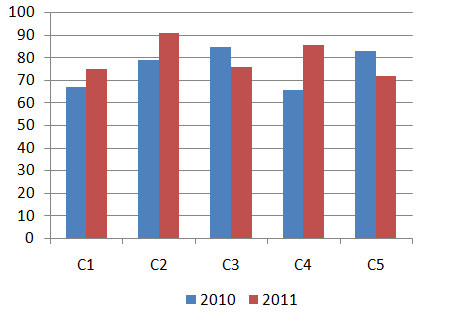Question 105

# Directions:Study the following bar graph and answer the question that follows:Sales of books (in thousands) from 5 (C1,C2,C3,C4 and C5) branches of a publishing company for the two successive years 2010 and 2011.What is the ratio of the total sales of branch C2 for both years to the total sales of branch C4 for both years?Solution

Total sales of branch C2 for both years (in thousands) = 79 + 91 = 170

Total sales of branch C4 for both years (in thousands) = 66 + 86 = 152

=> Required ratio = 170:152

=> Ans - (D)